# Active loads and the DP800, DP1000 series of Power Supplies

Posted on: August 20th, 2021 by James

Active loads and the RIGOL DP800 and DP1000 Series 1.

1. Introduction
The RIGOL DP800 series and DP1000 series are programmable linear DC power supplies. They can only provide power for a pure load that does not have the ability to output a current.
Active loads, such as those that can provide power (batteries, solar cells, etc..), should not be used with the DP800 series or DP1000 series power supply. Active loads can lead to instability in the power supply control loop and may damage the powered device.
Connecting the power supply to active loads is not recommended.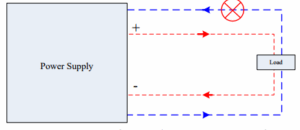Figure 1 Improper use of DP800 and DP1000 Series Power Supply

2. Detailed Technical Description
The RIGOL DP800 series and DP1000 series programmable linear DC power supply can only work in the first quadrant (source positive voltage and a positive current) or the third quadrant (source negative voltage and a negative current).
They cannot work in the second quadrant (negative voltage, positive current.. an adjustable load of negative power) or the fourth quadrant (positive voltage, negative current.. as used for a battery discharge test).
When the load itself is a source and the power supply is required to work in the second quadrant as an adjustable load, the control loop may lose control and the power supply will output an uncontrolled voltage. This could damage or destroy the load.

When the power supply works in the fourth quadrant (e.g., used in a battery discharge test), the control loop is also unstable and will quickly drain the battery. This can result in dangerous conditions, including damage to the battery, power supply, and a very high risk of fire and explosion.

The Cartesian coordinate system is a common representation of power supply output capabilities. The horizontal axis represents voltage, and the vertical axis represents current. The distributions of the four quadrants of the power supply as shown in Figure 2.
The first quadrant: the power supply provides a positive voltage and a positive current (the direction of the current flows from the power supply to the load).
The second quadrant: the power supply provides a negative voltage and a positive current (the direction of the current flows from the power supply to the load).
The third quadrant: the power supply provides a negative voltage and negative current (the direction of the current flows from the load to the power supply).
The fourth quadrant: the power supply provides a positive voltage and a negative current (the direction of the current flows from the load to the power supply).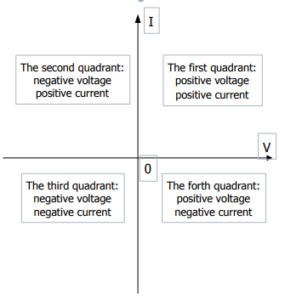Figure 2 Distributions of Power Quadrants

2.2.Principle of DP800 and DP1000
Here is a block diagram of DP800 and DP1000 series: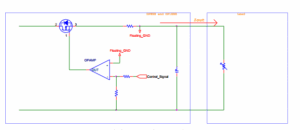Figure 3 Block Diagram of DP800 and DP1000

If a current is forced into the supply (I.E. sinking the current), it will directly affect the working status of the MOS transistor and result in instability within the control loop of the power supply as shown in Figure 4.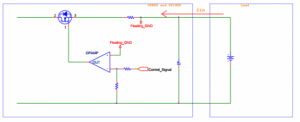Figure 4 Current Anti-irrigation Diagram

In addition, the DP800 and DP1000 series power supply outputs do not have an output relay. When a specific output channel is disabled (power off) the output voltage is set to 0V and is regulated by the control loop.

For charging batteries with the DP800 and DP1000 series power supplies, we recommend using constant current mode and implement the circuit shown in Figure 5. The external diode can prevent the flow of current into the supply and prevent damage.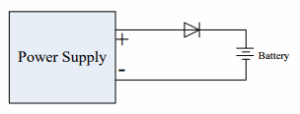Figure 5 Application Program of Battery Charge Test